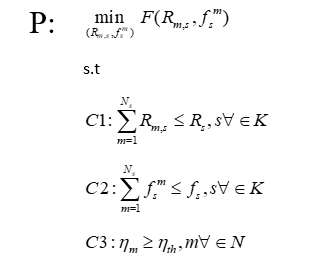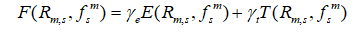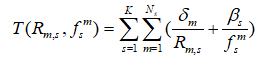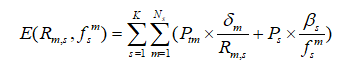# How solve this optimization problem using cvx solve?

Hi everyone! Am new in optimization solving problem, please any suggestion on how to use cvx solver to solve my snapshot problem? ThankswhereDo you understand, i mean really understand what your optimization problem specification is? Because I don’t. I see symbols which I don’t know what they are.

What is E? Is that some kind of mathematical expectation, or some function? What is T? It is incumbent on you to tell us exactly what it is, and to determine whether F is a joint;y convex function of its arguments. Copying an image out of a paper or book of an optimization problem specification which you don’t understand, is not going to be a good way to learn optimization.

Start off by reading this Why isn't CVX accepting my model? READ THIS FIRST! . And the CVX Users’ Guide http://cvxr.com/cvx/doc/ . But I’m afraid that you will have to learn something about optimization first, and this forum is not he place to do that.And if F is not a joint;y convex function of its arguments, you will not be able to use CVX to solve this problem.

Actually the objective function F is joint function between the energy(E) and time(T), my objective to minimize the energy together with the reducing the total execution time with limits of transmission rate R and frequency capability f. I have already define all the variables but to use cvx solver to solve my problem is challenge?

You have ti provide explicit formulas for E and T. Otherwise, no one on this forum will be able to help you.

F needs to be jointly convex in its argument. Otherwise, CVX can not be used for this problem. it is incumbent on you to prove (show) that tit is jointly convex in its arguments. That is addressed in the previously provided link, Why isn't CVX accepting my model? READ THIS FIRST! .

Thank you again
Definition of time and energyIf the numerators are nonnegative and the denominators can be constrained to be positive for both `P` and `E`, ( I don’t see such constraints in what you provided), then `inv_pos` can be used, inside of `sum(sum( ... ))` . Rather than my writing the code, I will require you to read the CVX User’s Guide (link in previous post) and try yourself, and ask for additional help only after you have done so.

If the numerators are not nonnegative or denominators can be constrained to be positive for both `P` and `E`, then the model can not be handled by CVX and is likely non-convex.

help inv_pos

inv_pos Reciprocal of a positive quantity.
inv_pos(X) returns 1./X if X is positive, and +Inf otherwise.
X must be real.

`````` For matrices and N-D arrays, the function is applied to each element.

Disciplined convex programming information:
inv_pos is convex and nonincreasing; therefore, when used in CVX
specifications, its argument must be concave (or affine).

Other functions named inv_pos``````

Thank you for the suggestions